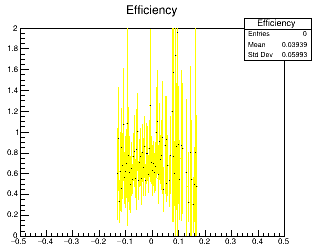# Average of a histogram

Hello,

I tried to use the following link. But it doesn’t help.

Thank you
DilHello,

GetMean is not what I expect.It doesn’t give me correct answer.
I need to add up the bin contents in the range
[-0.06,0.1] GeV2 and then divide by the number of bins from -0.06 to 0.1

Thanks
Dil

``````// assuming "h" is a pointer to your histogram
Int_t b1 = h->FindFixBin(-0.06);
Int_t b2 = h->FindFixBin(0.1);
Double_t v = h->Integral(b1, b2);
v /= b2 - b1 + 1;
std::cout << v << std::endl;
``````

Thank you. This worked.
h->GetMean() didn’t work. Because it
returns the average x-value of the histogram, not the mean of the y-values.

This topic was automatically closed 14 days after the last reply. New replies are no longer allowed.